Polymer Based PCF with High Nonlinearity and Low Bend Loss: A NewModeling | OMICS International
Journal of Lasers, Optics & Photonics

# Polymer Based PCF with High Nonlinearity and Low Bend Loss: A NewModeling

Muduli N*

Gandhi Engineering College, Bhubaneswar, Odisha, India

*Corresponding Author:
Muduli N
Gandhi Engineering College
Bhubaneswar, Odisha, India
Tel: 073810 54131
E-mail: [email protected]

Received date: January 09, 2017; Accepted date: January 31, 2017; Published date: February 15, 2017

Citation: Muduli N (2017) Polymer Based PCF with High Nonlinearity and Low Bend Loss: A New Modeling. J Laser Opt Photonics 4:147. doi: 10.4172/2469-410X.1000147

Copyright: © 2017 Muduli N. This is an open-access article distributed under the terms of the Creative Commons Attribution License, which permits unrestricted use, distribution, and reproduction in any medium, provided the original author and source are credited.

Visit for more related articles at Journal of Lasers, Optics & Photonics

#### Abstract

The effect of ellipticity ratio on low bend loss, high nonlinearity, wideband high birefringence and low confinement loss in a hexagonal spiral thermoplastic polymer based PCF having air holes with Kerr nonlinearity is investigated. Moreover, the above all property are simulated initially with wide range of wavelength (for different value ellipticity ratio) and secondly with ellipticity ratio at an operating wavelength of 1.55 μm. Through the optimization of arrangement and diameter of elliptical air holes, the designed spiral PCF offers low bend loss, high nonlinearity depends on mode field area (As small as mode field area, increase the nonlinearity), high birefringence and nonzero dispersion for X/Y polarization within the wavelength range of 0.5 to 1.75 μm. These results seem to be most useful in optical communication.

#### Keywords

Bend loss; High nonlinearity; Spiral PCF

#### Introduction

Photonic Crystal Fiber (PCF) consists of a large number of rectangular air holes running along the fiber, has gained much attention because it can be designed to yield many useful properties, such as endless single mode operation, flexible dispersion control, extremely high nonlinearity and birefringence. These properties can be explored for implementing new fiber devices for many applications. The main difference between PCF and conventional fiber is that photonic crystal fiber has air silica cross-section, whereas standard optical fiber has all glass cross-section . It has been shown that PCF possess different types of unique properties that are unachievable by conventional optical fibers, such as endlessly, single mode propagation , highly configurable dispersion, tailorable modal area  and birefringence . Several methods have been employed to study the characteristics of PCFs, including effective index method , the plane wave expansion method , the localized function method , the beam propagation method , the finite element method , and the finite difference time domain  or frequency domain method . Among these techniques, the FDTD method using Yee’s technique has been widely accepted as a very powerful computational technique for electromagnetic problem, and it is much easier to implement with comparable accuracy.

Although high birefringence, large nonlinearity, and low dispersion have been separately shown in different PCF structures, there has been little research reported in simultaneously achieving all of these features. In this scenario, a laudable goal would be to take full use of the design freedom of PCF to obtain simultaneous wideband high birefringence, large nonlinearity and nonzero dispersion which might be important in highly efficient wideband signal processing application. In addition, it is also desired to decrease the complexity of PCF design; and hence elliptical air holes with new tailoring arrangement are preferred.

Though this research paper is a new modeling for polymer based photonic crystal fiber and not compared with other research article, but some article has been worked out while considering single fiber cladding structure for investing bend loss, effective mode field area, confinement loss, bending sensing and mode coupling etc., for optical communication. For example, the ES PCF has excellent modal confinement properties, together with several parameters to allow the optimization of the performance over a range of important characteristics. This ES PCF design shows ultra low bending loss  and very low confinement loss and ability for very effective control of the modal field. The fabrication of the ES PCF should be possible using the extrusion and/or drilling. Similarly an index-guiding PCF  with an array of air holes surrounding the silica core region has special characteristics compared to conventional single-mode fibers. Using the effective index method and the Gaussian beam propagation theory, the macro-bending and splice losses for PCFs are investigated.

But this research article investigated on optimized proposed polymer based fiber design have studied the exceptional characteristics of low bend loss, high nonlinearity, high birefrigence and effective mode field area, low loss etc for optical communication. The bend loss calculation is done  and its mode field distribution profile is obtained using 2D FDTD technique. The proposed fiber structure is carefully fabricated by choosing the suitable parameter like air hole diameters (d1=diameter of major axis, d2=diameter of minor axis), pitch ? and operating wavelength in more accuracy. So this research article gives rise to an excellent result for above such property for optical communication.

In this article, we investigate a novel structure of thermoplastic polymer based Grade (III) Teflon (2400) PCF, where elliptical air holes are tailored in a hexagonal spiral array fashion. The above spiral PCF could offer low bend loss, small effective mode field area leads high nonlinearity, along with wideband high birefringence and low confinement loss for optical communication.

#### PCF Structure

We construct a novel spiral thermoplastic polymer based Grade (III) Teflon (2400) PCF by filling air holes at triangular position and numerically simulate the low bending loss, high nonlinearity and mode field area for optical communication purpose. The design structure of spiral PCF as illustrated in Figure 1b, i.e., similar to interesting spiral phenomenon in nature like sun flower seeds as shown in Figure 1a. Our design PCF hexagonal core with different spiral is composed of elliptical air holes. In order to obtain low bend loss and high nonlinearity, a spiral polymer based PCF is to be chosen. The structure has eight spiral arms, each composed of three elliptical air holes with different diameter. The all spiral arms occur with changing bending orientation and changeable spacing between elliptical air holes. In our PCF design the arc distance between the neighboring air hole is same. We calculate the field distribution; bend loss, high nonlinearity, effective mode field area and confinement loss of the designed polymer spiral PCF by using 2D FDTD method. The spiral lattice of elliptically air holes are distributed in thermoplastic polymer based PCF (Figures 1a and 1b).

#### Numerical Analysis

To study the field distribution of hexagonal spiral thermoplastic polymer like Grade (III) Teflon (2400) PCF, we have used 2-dimensional finite difference time domain (FDTD) method. Considering the material is isotropic, linear, and lossless, the time dependent Maxwell’s equations can be written as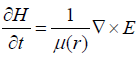(1)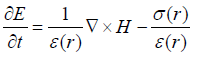(2)

E and H are electric field and magnetic field.

Where ε(r), μ(r), σ(r) are permittivity, permeability and conductivity of the material and all are in the function of position.

Equations (1) and (2) can be discretized using Lee’s technique. Considering spatial and time discretization, equations (1) and (2) can be written for TE polarization as follows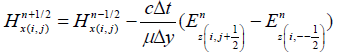(3)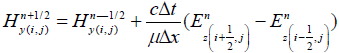(4)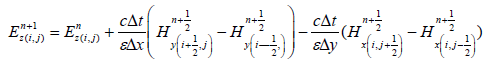(5)

For stability, the time step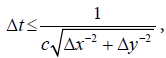where Δt the time increment, c is the velocity of light, Δx be the lattice increment in x direction, Δy be the lattice increment along y direction.

Considering equation (3), (4) and (5), we have calculated the field distribution of PCFs in TE polarization mode.

For a nonlinear optical waveguide having Kerr-type non-linearity related permittivity εr depends on electric field Ey and can be expressed as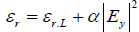(6)

Where εrL is the linear relative permittivity and α is the non linear co-efficient. A hybrid implicit FDTD method is used to simulate the field for 2D PCS with nonlinear rods. The overall stability of this hybrid FDTD scheme is determined by the stability in the linear medium regions. Consequently, nonlinearity in the structure does not affect stability and hence the grid size and time step.

The nonlinear coefficient of PCF and dispersion control are rested with the design of the cladding structure parameters that is flexibility and comparative freedom, the cladding structure parameters mostly include air hole diameters( d1,d2), pitch ? and air filling rate ‘f’ of cladding. The nonlinear coefficient γ(λ) of PCF can be expressed as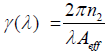(7)

Where Aeff is effective mode field area and n2=3.4 × 10-20 m2/W, is the nonlinear index of Grade (III) Teflon (AF2400).

In order to investigate the optical properties of proposed spiral PCF, we have given stress for dispersion property and the confinement loss of the same optical fiber. The chromatic dispersion plays an important role in optical communication is calculated as: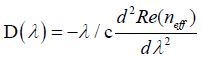(8)

Where Re (neff) is the real part of the refractive index in cladding.

The confinement loss of optical fiber can be calculated using fundamental formula, which is given below.

Confinement loss=8.686k0Im [neff] dB/km    (9)

Similarly, effective mode field area can be expressed as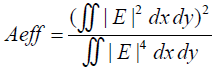(10)

This integration is calculated over the cross-section of the fiber and the electric field intensity E is given by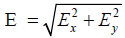(11)

Where Ex is the x-component of the electric field and Ey is the y-component of the electric field.

The bend loss in photonic crystal fiber can be calculated as: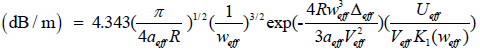Where R=bend radius, aeff=effective mode field area, K1(weff) is the modified Bessel first kind, Δeff=relative difference between the core and effective cladding indices, Veff=effective normalized frequency and Ueff is the another waveguide parameter respectively.

#### Results and Discussion

We investigate the field profile pattern and effective refractive index of the designed spiral PCF by implementing 2D FDTD technique. The spiral lattices of elliptical air holes are distributed in a Grade (III) Teflon polymer material taken into account. However the nonlinear refractive index of above PCF is about 3.4 × 10-20 m2/W considered using Sellemier equation. We also report the dispersion property, low loss, small MFA and high nonlinearity of above spiral PCF design in accurate way. The above all property studied with wide range of wavelength for different ellipticity ratio and secondly with ellipticity ratio at an operating wavelength (1.55 μm) of incident light used (Figures 2a and 2b).

Figure 2: (a) dispersion versus wavelength for different value of ellipticity ratio and (b) dispersion versus ellipticity value for operating wavelength of 1.55 μm.

To ensure accuracy report, Figure 2a represents the chromatic dispersion versus range of wavelength of different values of ellipticity. It reveals that dispersion property gradually increase with increase of wavelength and found that increasing the value of ellipticity which extend increase the dispersion property while considering dispersion versus ellipticity value at an operating wavelength of 1.55 μm. It is seen from Figure 2b that dispersion property exponentially increases with increase in ellipticity ratio. In contrast to the conventional single mode fiber, the above spiral PCF provides increasing freedom in tailoring birefringence nonlinearity and effective mode field area. It has been reported that PCF can realize endlessly single mode operation, high group of birefringence, flexible chromatic dispersion over a wide wavelength range, large effective area etc. Among the features of PCFs, birefringence is one of the most interesting characteristics. Birefringence in PCFs usually results intentionally reducing the rotational symmetry of the fiber structure. The effect of the birefringence is more obvious, if the index contrast between the core cladding is high. A high level of birefringence is often required in many sensing applications and in applications where light is required to maintain a linear polarization state. The most common technique to break the PCF structural symmetry is by changing the air hole sizes along two orthogonal axes near the core region. We first report the dependence of birefringence on range of wavelength for different values of ellipticity and secondly on range of ellipticity ratio of air hole at an operating wavelength of 1.55 μm (Figures 3a and 3b).

Figure 3: (a) Birefringence versus wavelength for different value of ellipticity. (b). Birefringence versus ellipticity ratio for operating wavelength.

The Figure 3a plots the variation of modal birefringence versus wavelength and it is found to report that birefringence property increase with increase of wavelength of input light, but some variation observed that birefringence is more for low value of ellipticity and low value for high value of ellipticity and it studied that birefringence exponentially decrease with increase in ellipticity value shown in Figure 3b and it may also clearly seen that the same property increase with increase in the diameter of air holes. The Figure 3b depicts the field distribution of FMs for x/y polarization under two different diameters of air hole at operating wavelength of 1.55 μm.

The high nonlinear coefficient and designable dispersion properties make these fibers attractive for many nonlinear applications of which super continuum (SC) generation has been the most intensively investigated and this has been used in application like optical coherence tomography, spectroscopy, metrology and wavelength division multiplexing etc. High nonlinear PCFs are good approach and used to generate super continuum generation pumped by ultrafast laser pulses and longer laser pulses, however super continuum generation in PCFs is restricted by dispersion properties. Mode field area of PCF is the key factor to generate the nonlinear coefficient i.e. as small as the mode field area, the increase in the nonlinear coefficient which leads to super continuum generation. We further characterize the performance of mode field area nonlinearity and low loss hexagonal spiral PCF. In fact nonlinear coefficient attains high value for low values of mode field area Figures 4a and 4b).

Figure 4: (a) Non linear coefficient versus wavelength for different wavelength and (b) Nonlinear coefficient versus ellipticity ratio for operating wavelength of 1.55 μm.

It can clearly indicate in Figure 4a the nonlinear coefficient exponentially decreases with increasing in wavelength of incident light. However for low value of ellipticity ratio 1.2, nonlinear coefficient attains high value. Moreover, nonlinear coefficient exponentially decreases with increase in ellipticity ratio. Nonlinear coefficient may lead to the study of supercontinuum generation.

Similarly, another variation between mode field area versus range of wavelength and ellipticity ratio demonstrated in Figures 5a and 5b. Initially Figure 5a demonstrates that the variation between mode field area versus wavelength for different value of ellipticity and clearly observed that Aeff approximate expontially increase with increasing in wavelength and achieve low value for small value of ellipticity and attaim maximum value for high value of ellipticity. However, Figure 5b shows that Aeff exponentially increase with rise in ellipticity. It is reported for Figures 2-5 that hexagonal spiral PCF can provide simultaneous wide band high birefringence, large nonlinearity and low dispersion. The proposed structure employs spiral arranged elliptical air holes. In contrast to conventional PCF structure supporting separate property (Figures 6a and 6b).

Figure 5: (a) Mode field area versus wavelength for different ellipticity value and (b) Mode field area versus ellipticity ratio for operating wavelength of 1.55 μm.

PCFs are single material fibers with an arrangement of air holes running along the length of fiber and they provide confinement and guidance of light in a defect region around the centre. More over low loss in PCF is more significant contribution for optical property, therefore, Figures 6a and 6b plot the variation of confinement loss versus wavelength range and also with ellipticity ratio. It is seen from Figure 6a that confinement loss decrease gradually with increase in wavelength of incident light used and it has more loss for ellipticity 2.4 and low loss for ellipticity 1.2. Furthermore, analyzed that confinement loss increase with increase of ellipticity in the order of 10-2 and operating at a wavelength of 1.55 μm. Since bend loss in PCF can be controlled by varying fiber parameter and it reveals that the minimum bend loss increase as the air hole size becomes large at an operating wavelength of 1.55 μm range and hence it expects that this study will be helpful in the design of PCF with bend loss for a broad spectral range (Figures 7a and b).

We next studied the relation between bend loss versus wide range of wavelength and as well as with ellipticity ratio at an operating wavelength of 1.55 μm shown in Figures 7a and 7b. The results found that bend loss exponentially decrease (in the order of 10-3) with increase in wavelength of incident light, furthermore analyze that bend loss gradually decrease with decrease in ellipticity ratio, and is simulated at an operating wavelength of 1.55 μm. This results show that the small difference in bend loss for different values of ellipticity ratio or the use of temperature sensitive stress may prevent single mode operation in high power application.

#### Conclusion

In summary, a simple novel the thermoplastic polymer based PCF has been proposed. Guiding properties like low bend loss, high nonlinearity, effective index of core and cladding and dispersion are numerically investigated by 2D FDTD technique. The effect of ellipticity ratio and diameter of circular air holes on PCF structure may cause the wideband birefringence, high nonlinearity dispersion and confinement loss. The spiral PCF offers high birefringence in the order of 10-5, small effective area may leads to high nonlinearity of approximate 60 W-1. km-1 and confinement loss is in the order of 10-2.

#### References

Select your language of interest to view the total content in your interested language

### Article Usage

• Total views: 1105
• [From(publication date):
March-2017 - Dec 15, 2019]
• Breakdown by view type
• HTML page views : 1009Can't read the image? click here to refresh